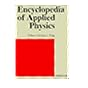Normal view MARC view ISBD view

# Encyclopedia of Mathematical Physics. Volume 5. St-Z.REF/HAND DR (v. 9) Encyclopedia of applied physics, volume 9, Magnetic materials to mechanical vibration and damping Ref/Hand ENC Encyclopedia of mathematical physics. Volume 2: D-H Ref/Hand ENC Encyclopedia of mathematical physics. Volume 1. A-C. Ref/Hand ENC Encyclopedia of Mathematical Physics. Volume 5. St-Z. REF/HAND ENC Encyclopedia of Mathematical Physics. Volume 3. I-O. Ref/Hand ENC Encyclopedia of Mathematical Physics. Volume 4. P-Sp. Ref/Lil DR Quantities, units and symbols, Second edition